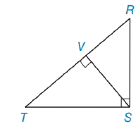Chapter 5.4, Problem 2EElementary Geometry For College St...

7th Edition
Alexander + 2 others
ISBN: 9781337614085

Solutions

Chapter
SectionElementary Geometry For College St...

7th Edition
Alexander + 2 others
ISBN: 9781337614085
Textbook Problem

Use theorem 5.4.2 to form a proportion in which SV is a geometric mean.[Hint: Δ S V T ∼ Δ R V S ]Exercises 1-6

To determine

To Find:

Proportion in which SV is the geometric mean.

Explanation

Given figure,

From the figure,

ΔRST is a right triangle with right angle RST, and SV¯ is the altitude to hypotenuse TR¯.

From the theorem,

The length of the altitude to the hypotenuse of a right triangle is the geometric mean of the lengths of the segments of the hypotenuse.

Since ΔRVSΔSVT

Corresponding sides of similar triangles are proportional(CSSTP)

So, we have

VTSV=SVRV

Recall the Means-Extremes Property

In a proportion, the product of the means equals the product of the extremes

Still sussing out bartleby?

Check out a sample textbook solution.

See a sample solution

The Solution to Your Study Problems

Bartleby provides explanations to thousands of textbook problems written by our experts, many with advanced degrees!

Get Started

let f(x) = x3 + 5, g(x) = x2 2, and h(x) = 2x + 4. Find the rule for each function. 6. fgh

Applied Calculus for the Managerial, Life, and Social Sciences: A Brief Approach

Elimination of the parameter in x = 2t3/2, y = t2/3 gives: x4 = 16y9 16x4 = y4 x3 = 8y4 8x3 = y4

Study Guide for Stewart's Single Variable Calculus: Early Transcendentals, 8th

The unit vector in the direction of is:

Study Guide for Stewart's Multivariable Calculus, 8th